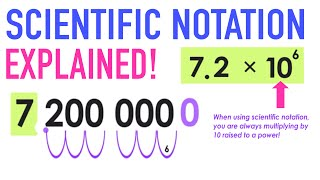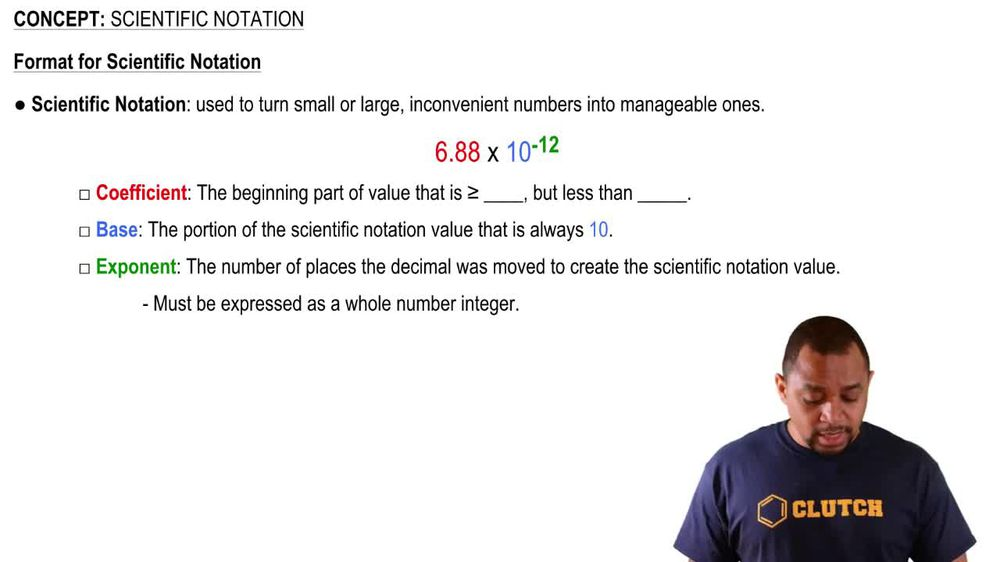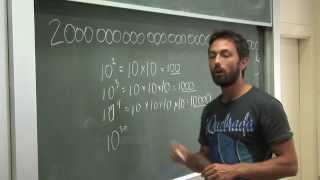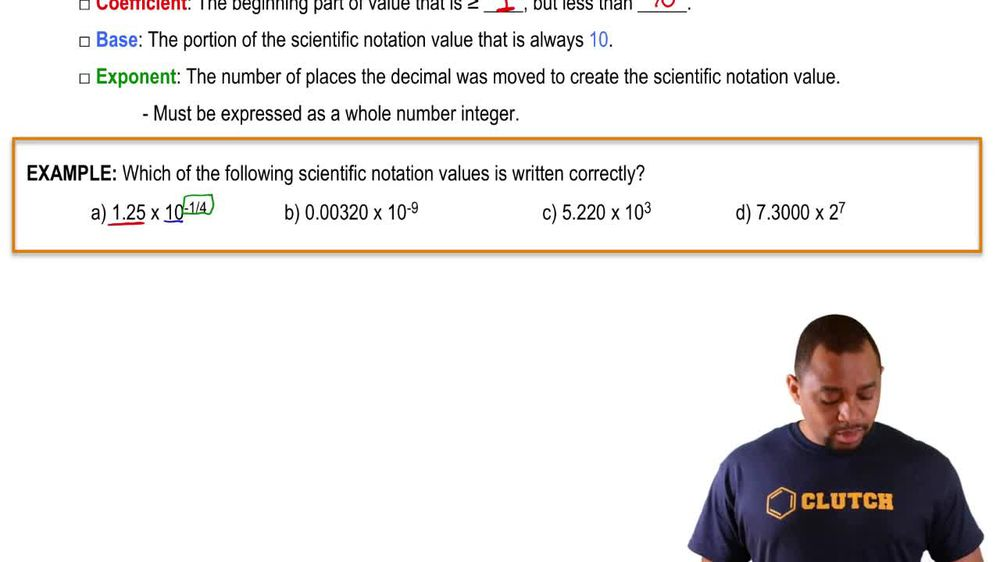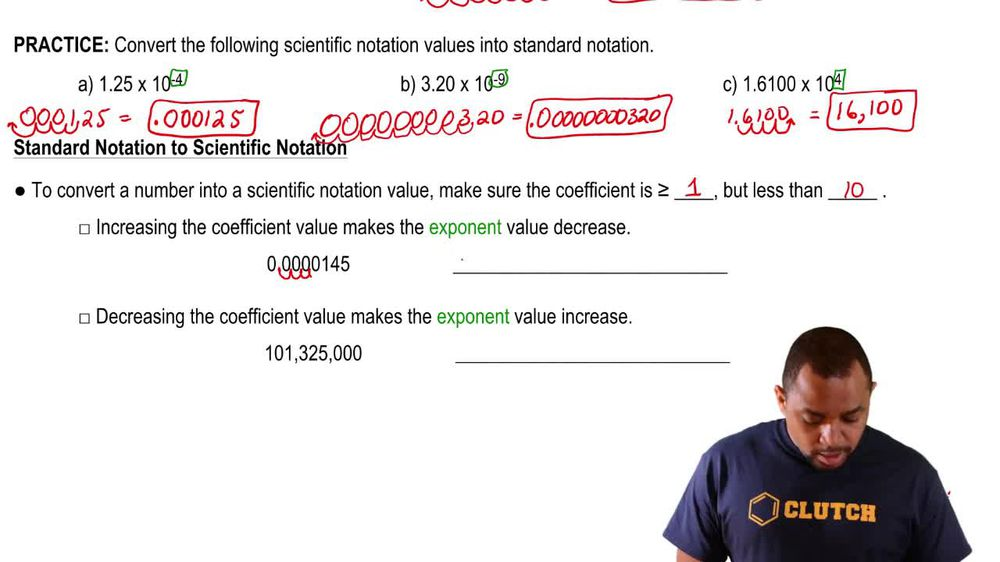Start typing, then use the up and down arrows to select an option from the list.
1. 1. Intro to General Chemistry2. Scientific Notation
Problem

# Express the following measurements in scientific notation. (a) 453.32 mg

Relevant Solution56s
Play a video:
Hi everyone here. We have a question telling us that the elevation of Mount Everest is 8848.86 m. And our goal here is to express this in scientific notation with two significant figures. So let's write this back out .86 m. So two significant figures means we are stopping at this eight here, so we want to move our decimal place 12 three places to the left, So that will be 8.8 times 10 to the third. And that is our final answer. Thank you for watching. Bye.